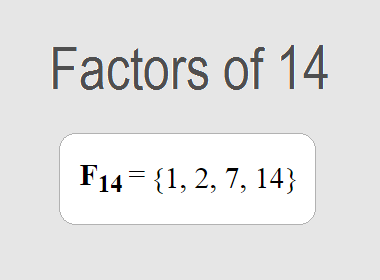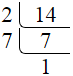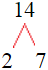# Factors of 14The factors of 14 are 1, 2, 7, and 14 i.e. F14 = {1, 2, 7, 14}. The factors of 14 are those numbers that can divide 14 without leaving a remainder.

We can check if these numbers are factors of 14 by dividing 14 by each of them. If the result is a whole number, then the number is a factor of 14. Let's do this for each of the numbers listed above:

·        1 is a factor of 14 because 14 divided by 1 is 14.

·        2 is a factor of 14 because 14 divided by 2 is 7.

·        7 is a factor of 14 because 14 divided by 7 is 2.

·        14 is a factor of 14 because 14 divided by 14 is 1.

## How to Find Factors of 14?

1 and the number itself are the factors of every number. So, 1 and 14 are two factors of 14. To find the other factors of 14, we can start by dividing 14 by the numbers between 1 and 14. If we divide 14 by 2, we get a remainder of 0. Therefore, 2 is a factor of 14. If we divide 14 by 3, we get a remainder of 2. Therefore, 3 is not a factor of 14.

Next, we can check if 4 is a factor of 14. If we divide 14 by 4, we get a remainder of 2. Therefore, 4 is not a factor of 14. We can continue this process for all the possible factors of 14.

Through this process, we can find that the factors of 14 are 1, 2, 7, and 14. These are the only numbers that can divide 14 without leaving a remainder.

********************

********************

## Properties of the Factors of 14

The factors of 14 have some interesting properties. One of the properties is that the sum of the factors of 14 is equal to 24. We can see this by adding all the factors of 14 together:

1 + 2 + 7 + 14 = 24

Another property of the factors of 14 is that they are all prime numbers except 1 and 14.

## Applications of the Factors of 14

The factors of 14 have several applications in mathematics. One of the applications is in finding the highest common factor (HCF) of two or more numbers. The HCF is the largest factor that two or more numbers have in common. For example, to find the HCF of 14 and 21, we need to find the factors of both numbers and identify the largest factor they have in common. The factors of 14 are 1, 2, 7, and 14. The factors of 21 are 1, 3, 7, and 21. The largest factor that they have in common is 7. Therefore, the HCF of 14 and 21 is 7.

Another application of the factors of 14 is in prime factorization. Prime factorization is the process of expressing a number as the product of its prime factors. The prime factors of 14 are 2 and 7, since these are the only prime numbers that can divide 14 without leaving a remainder. Therefore, we can express 14 as:

14 = 2 × 7

We can do prime factorization by division and factor tree method also. Here is the prime factorization of 14 by division method,14 = 2 × 7

Here is the prime factorization of 14 by the factor tree method,14 = 2 × 7

## Conclusion

The factors of 14 are the numbers that can divide 14 without leaving a remainder. The factors of 14 are 1, 2, 7, and 14. The factors of 14 have some interesting properties, such as having a sum of 24. The factors of 14 have several applications in mathematics, such as finding the highest common factor and prime factorization.